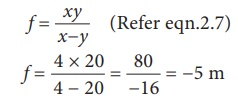Home | | Science 10th Std | Solved Problems: Optics

# Solved Problems: Optics

Science : Optics: Solved Problems, Solved Example Problems with Answers, Solution

Optics Science

SOLVED PROBLEMS

### Problem 1

Light rays travel from vacuum into a glass whose refractive index is 1.5. If the angle of incidence is 30┬░, calculate the angle of refraction inside the glass.

### Solution:

accorting to SnellŌĆÖs law,

sin i / sin r = ┬Ą2/ ┬Ą1

┬Ą1 sin i = ┬Ą2 sin r

Here ┬Ą1 = 1.0,

┬Ą2 = 1.5, i = 30┬░

(1.0) sin 30┬░ = 1.5 sin r

1├Ś 1/2 = 1.5 sin r

sin r = 1/(2├Ś1.5) = 1/3 = (0.333)

r= sinŌĆō1 (0.333)

r = 19.45┬░

### Problem-2

A beam of light passing through a diverging lens of focal length 0.3m appear to be focused at a distance 0.2m behind the lens. Find the position of the object.

### Solution:

f = ŌłÆ0.3 m, v = ŌłÆ0.2 m### Problem-3

A person with myopia can see objects placed at a distance of 4m. If he wants to see objects at a distance of 20m, what should be the focal length and power of the concave lens he must wear?

### Solution:

Given that x = 4m and y = 20m.

Focal length of the correction lens is

f = ŌĆēŌĆēŌĆēxy / ŌĆēxŌłÆyŌĆē (Refer eqn.2.7)Power of the correction lens### Problem-4

For a person with hypermeteropia, the near point has moved to 1.5m. Calculate the focal length of the correction lens in order to make his eyes normal.

### Solution:

Given that, d = 1.5m; D = 25cm = 0.25m (For a normal eye).

From equation (2.8), the focal length of the correction lens is

f = ŌĆēd ├Ś D / ŌĆēd ŌłÆ D = ŌĆē1.5 ├Ś 0.25 / 1.5 ŌłÆ 0.25 = ŌĆē0.375 /ŌĆē1.25 = 0.3 m

Tags : Science , 10th Science : Chapter 2 : Optics
Study Material, Lecturing Notes, Assignment, Reference, Wiki description explanation, brief detail
10th Science : Chapter 2 : Optics : Solved Problems: Optics | Science